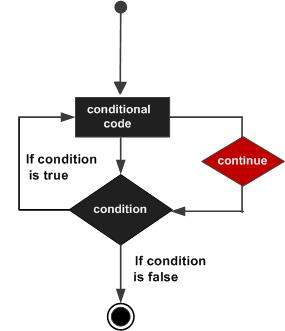# C++ continue statement

#### C in Depth: The Complete C Programming Guide for Beginners

45 Lectures 4.5 hours

#### Practical C++: Learn C++ Basics Step by Step

Most Popular

50 Lectures 4.5 hours

#### Master C and Embedded C Programming- Learn as you go

66 Lectures 5.5 hours

The continue statement works somewhat like the break statement. Instead of forcing termination, however, continue forces the next iteration of the loop to take place, skipping any code in between.

For the for loop, continue causes the conditional test and increment portions of the loop to execute. For the while and do...while loops, program control passes to the conditional tests.

## Syntax

The syntax of a continue statement in C++ is −

```continue;
```

## Flow Diagram## Example

```#include <iostream>
using namespace std;

int main () {
// Local variable declaration:
int a = 10;

// do loop execution
do {
if( a == 15) {
// skip the iteration.
a = a + 1;
continue;
}
cout << "value of a: " << a << endl;
a = a + 1;
}
while( a < 20 );

return 0;
}
```

When the above code is compiled and executed, it produces the following result −

```value of a: 10
value of a: 11
value of a: 12
value of a: 13
value of a: 14
value of a: 16
value of a: 17
value of a: 18
value of a: 19
```
cpp_loop_types.htm